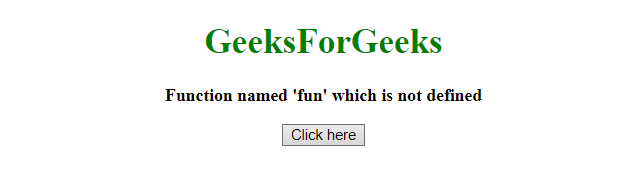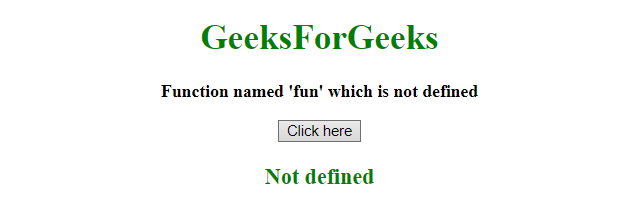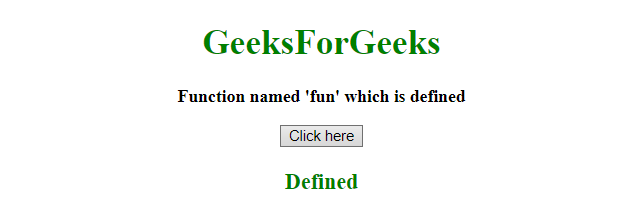# How to check a function is defined in JavaScript ?

The job is to identify whether a function is defined or not. The JavaScript typeof operator is used to solve the problem described below:

Javascript typeof Operator: This operator can be used to find the type of a JavaScript variable. This operator returns the type of a variable or an expression:

Syntax:

`typeof var`

Parameter: It contains single value var which is a Javascript variable.

Return value: It returns the type of a variable or an expression:

Example 1: This example checks the type of the function, If it is function then it is defined otherwise not defined by using typeof operator.

 `  ` `<``html``>  ` `    ``<``head``>  ` `        ``<``title``>  ` `            ``How to check a function is defined ` `        `` ` `    ``  ` `     `  `    ``<``body` `style` `= ``"text-align:center;"``>  ` `     `  `        ``<``h1` `style` `= ``"color:green;"` `>  ` `            ``GeeksForGeeks  ` `        ``  ` `         `  `        ``<``p` `id` `= ``"GFG_UP"` `style` `= ``"font-size: 16px; font-weight: bold;"``> ` `        `` ` `         `  `        ``<``button` `onclick` `= ``"gfg_Run()"``>  ` `            ``Click here ` `        `` ` `         `  `        ``<``p` `id` `= ``"GFG_DOWN"` `style` `=  ` `            ``"color:green; font-size: 20px; font-weight: bold;"``> ` `        `` ` `         `  `        ``<``script``> ` `            ``var el_up = document.getElementById("GFG_UP"); ` `            ``var el_down = document.getElementById("GFG_DOWN"); ` `             `  `            ``el_up.innerHTML =  ` `                ``"Function named 'fun' which is not defined"; ` `                 `  `            ``function gfg_Run() { ` `                ``var defined = 'Not defined'; ` `                ``if(typeof fun == 'function') { ` `                    ``defined = "Defined"; ` `                ``} ` `                ``el_down.innerHTML = defined; ` `            ``}          ` `        ``  ` `    ``  ` `                     `

Output:

• Before clicking on the button:• After clicking on the button:Example 2: This example checks the type of the function, If it is function then it is defined otherwise not defined by using typeof operator by creating a function.

 `  ` `<``html``>  ` `    ``<``head``>  ` `        ``<``title``>  ` `            ``How to check a function is defined ` `        `` ` `    ``  ` `     `  `    ``<``body` `style` `= ``"text-align:center;"``>  ` `     `  `        ``<``h1` `style` `= ``"color:green;"` `>  ` `            ``GeeksForGeeks  ` `        ``  ` `         `  `        ``<``p` `id` `= ``"GFG_UP"` `style` `= ``"font-size: 16px; font-weight: bold;"``> ` `        `` ` `         `  `        ``<``button` `onclick` `= ``"gfg_Run()"``>  ` `            ``Click here ` `        `` ` `         `  `        ``<``p` `id` `= ``"GFG_DOWN"` `style` `=  ` `            ``"color:green; font-size: 20px; font-weight: bold;"``> ` `        `` ` `         `  `        ``<``script``> ` `            ``var el_up = document.getElementById("GFG_UP"); ` `            ``var el_down = document.getElementById("GFG_DOWN"); ` `             `  `            ``el_up.innerHTML = "Function named 'fun' which is defined"; ` `             `  `            ``function isFunction(possibleFunction) { ` `                ``return typeof(possibleFunction) === typeof(Function); ` `            ``} ` `             `  `            ``function fun() { ` `                 `  `            ``} ` `             `  `            ``function gfg_Run() { ` `                ``var defined = 'Not defined'; ` `                ``if(isFunction(fun)) { ` `                    ``defined = "Defined"; ` `                ``} ` `                ``el_down.innerHTML = defined; ` `            ``}          ` `        ``  ` `    ``  ` `                     `

Output:

• Before clicking on the button:• After clicking on the button:• <

My Personal Notes arrow_drop_upCheck out this Author's contributed articles.

If you like GeeksforGeeks and would like to contribute, you can also write an article using contribute.geeksforgeeks.org or mail your article to contribute@geeksforgeeks.org. See your article appearing on the GeeksforGeeks main page and help other Geeks.

Please Improve this article if you find anything incorrect by clicking on the "Improve Article" button below.